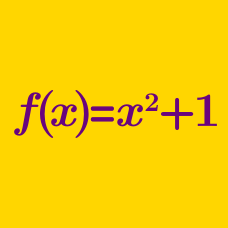Algebra

# Functions: Level 4 Challenges

Andrew has a favorite function $$A(x)=px+q^x$$ such that $$A(1)=4$$ and $$4A(2)=37$$, find the maximum value of $$p-q.$$

###### Try Part 1.

Suppose $$f$$ is a continuous, positive real-valued function such that $$f(x + y) = f(x)f(y)$$ for all real $$x,y.$$

If $$f(8) = 3$$ then $$\log_{9}(f(2015)) = \dfrac{a}{b}$$, where $$a$$ and $$b$$ are positive coprime integers. Find $$a - b.$$

$\begin{eqnarray} e(x)+o(x) &= & f(x) \\ e(x)+x^2& =& o(x) \\ \end{eqnarray}$ If the above two equations are true for every real $$x$$ where $$e(x)$$ and $$o(x)$$ are any even and odd functions respectively whereas $$f(x)$$ may be an ordinary function. Find $$f(2).$$

The lovely function $$f_{}^{}$$ has the beautiful property that, for each real number $$x$$:

$\large f(x)+f(x-1) = x^2.$

If $$f(19)=94$$, what is $$f(94)$$?

###### This is original.

$\begin{eqnarray} f_1 (x)&=&x\\ f_2 (x)&=&1-x\\ f_3 (x)&=&\frac{1}{x}\\ f_4 (x)&=&\frac{1}{1-x}\\ f_5 (x)&=&\frac{x}{x-1}\\ f_6 (x)&=&\frac{x-1}{x}\\ \end{eqnarray}$

If we know that $$f_6 (f_m(x))=f_4(x)$$ and $$f_n (f_4(x))=f_3(x)$$, find the minimum value of $$m+n$$.

×